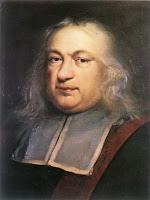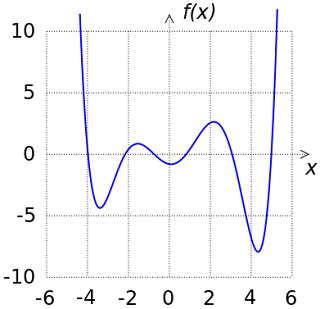## Monday, 15 April 2013

### The Key To Public Key Encryption

I've talked several times about how quantum computers could cause a problem for RSA based public key encryption as, using Shor's algorithm, you can theoretically factor large numbers in timescales short enough to render the encryption pointless.  However, conventional computers can be used to factor.  There are several algorithms that can be implemented.  Below I've tried to flag up the main algorithms that have been developed.

First, let's revisit the RSA algorithm. In RSA each user generates a key pair (one public and one private).  This is done by:Graph of GCDs
1. Randomly select two large prime numbers: p and q;
2. Calculate the modulus: n=p*q
3. Randomly choose an encryption key e such that 1 < e < φ(n) where the greatest common divisor (gcd) of e and φ(n) = 1 and φ(n) = (p-1)(q-1) where φ(n) is known as the Euler's totient.
From this you can find the decryption key d by solving the equation: -e * d = 1 (mod φ(n)) and 0 ≤ ≤ n. Then the user publishes their encryption key as {e, n} and keeps the decryption key {d, p, q} secret.First 1000 Values of φ(n)
For Alice to encrypt a message m to Bob, she needs only {e, n} and then computes c=me (mod n) where
0 ≤ m < n and Bob needs then only to compute m = cd (mod n).

To attack RSA you typically take one of three approaches:

1. Factor n = p*q and hence compute φ(n) and thence d; or
2. Determine φ(n)  directly (without factoring) and thence d; or
3. Determine d directly
Current opinion suggests (although I am not aware of any proofs) that each is as computationally difficult as the other. It is factoring that has the greatest number of algorithms as they have evolved over the entire history of mathematics not just since its use in cryptography.

The factoring algorithms general considered are when factoring large numbers for attacks on RSA are:Pierre de Fermat
1. Fermat's difference of squares: this dates from 1643. We find that any composite n = r * s can be expressed as  [(s+r)/2]2  - [(s-r)/2]2 from which Fermat showed that by calculating y2 - n using a specific sequence of y based upon n (easily calculated), you will find eventually find a perfect square from which you find s and r. The maximum number of steps needed to find the perfect square is (n - 2)/2 minus the largest integer less than or equal to square root of n
2. Euler's factoring method: Euler extended Fermat's method.  Euler noted that for some n two squares were equivalent in modulo n.  This allowed Euler to modify Fermat's approach slightly and sieve the results to reduce the number of values examined.
3. Kaitchik's method: as late as 1945 Kraitchik revisited Fermat's approach and found a further representation where the difference of squares was a multiple of n,  allowing further sieving of results. It is the basis of the quadratic sieve.Leonhard Euler
4. Pollard's P-1 method: this relies upon the fact that for one of the primes p, the number p -1 has only small prime factors. An RSA designer would try to avoid this situation so as to avoid Pollard's attack but often the primes are chosen randomly and this check may not be made.
5. Pollard's rho method: published just one year after his p -1 method, the rho method was published. It is based upon the Floyd cycling algorithm, also called the tortoise and hare algorithm as it has two pointers which sieve through the results but at different speeds.  It also uses the fact that for larger numbers there is a greater than 50% chance that numbers can have a form of equivalence in modulo arithmetic when there is a particular relationship between the numbers, rather like the idea of "collisions" that leads to the birthday paradox.An Early "Sieve" For Finding Primes
6. Quadtratic sieve: this was developed in the 1980s. It essentially a means of trying to set up equivalences of squares in modulo n plus a means of storing primes so that hey can be compared rapidly.It does rely upon a type of trial an error but stores useful results for comparison later in the algorithm.
7. General number field sieve: this s fastest factoring algorithm we currently have. It relies upon choosing polynomials  that have a common root related to n.  The efficiency of the algorithm depends very much on the choice of polynomials and there no known method for optimally choosing these.  Hence, whilst the quadratic sieve can be the fastest algorithm it is not always reliably so.  Hence, the quadratic sieve is often used inside.6th Order Polynomial
There is still much work to be done on factoring large numbers into constituent primes.  It is deeply mathematical but it does have some profound consequences for public key encryption.  Hence, although you may not be interested in understanding the detail, it is worth having an appreciation of what techniques continue to be developed in factoring large numbers.

Although it has been studied for hundreds of years, and despite our modern computing power, for numbers more than 100 bits there is still scope for improvement in algorithm design.  Research continues to be published and will continue I believe until we have access to a working quantum computer.